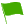Decimal Operations (SHSAT Level):

TIPS:

1. Addition: – line up addition and subtraction calculations vertically.
2. Multiplication – convert every decimal to a whole number and multiply. Add the number of digit places to the right of the decimal points for every number included in the product. Add back that total number of decimal places to the whole number answer.
3. Division – Move the decimal places in the denominator to make it a whole number. Move the decimal places in the numerator by the same amount and then divide.
4. Complex – Convert each decimal to a whole number multiplied by a power of 10. Make the appropriate calculations on the whole numbers and the powers of 10 separately (easier to simplify and compute). Convert the result back into a decimal format.
1.0.045/0.0015 = ?
A.
B.
C.
D.

Question 1 of 5

2.0.027/30 = ?
A.
B.
C.
D.

Question 2 of 5

3.3.6 ÷ 2/3 = ?
A.
B.
C.
D.

Question 3 of 5

4.5.44 + 5.46

Consider the following two methods to estimate the sum above:

Method I: Round each number to the nearest tenth, then add.

Method II: Round each number to the nearest whole number, then add.

By how much would the result of Method I be greater than the result of Method II?

A.
B.
C.
D.
E.

Question 4 of 5

5.50 × 0.033 = ?
A.
B.
C.
D.

Question 5 of 5